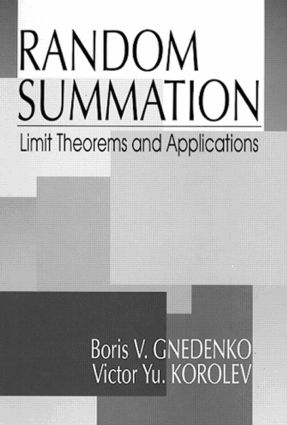Random Summation

Limit Theorems and Applications, 1st Edition

CRC Press

288 pages

Hardback: 9780849328756
pub: 1996-03-27
x

FREE Standard Shipping!

Description

This book provides an introduction to the asymptotic theory of random summation, combining a strict exposition of the foundations of this theory and recent results. It also includes a description of its applications to solving practical problems in hardware and software reliability, insurance, finance, and more. The authors show how practice interacts with theory, and how new mathematical formulations of problems appear and develop.

Attention is mainly focused on transfer theorems, description of the classes of limit laws, and criteria for convergence of distributions of sums for a random number of random variables. Theoretical background is given for the choice of approximations for the distribution of stock prices or surplus processes. General mathematical theory of reliability growth of modified systems, including software, is presented. Special sections deal with doubling with repair, rarefaction of renewal processes, limit theorems for supercritical Galton-Watson processes, information properties of probability distributions, and asymptotic behavior of doubly stochastic Poisson processes.

Random Summation: Limit Theorems and Applications will be of use to specialists and students in probability theory, mathematical statistics, and stochastic processes, as well as to financial mathematicians, actuaries, and to engineers desiring to improve probability models for solving practical problems and for finding new approaches to the construction of mathematical models.

Examples

Examples Related to Generalized Poisson Laws

A Remarkable Formula of Queueing Theory

Other Examples

Doubling with Repair

Mathematical Model

A Limit Theorem for the Trouble-Free Performance Duration

The Class of Limit Laws

Some Properties of Limit Distributions

Domains of Geometrical Attraction of the Laws from Class c

Limit Theorems for "Growing" Random Sums

A Transfer Theorem. Limit Laws

Necessary and Sufficient Conditions for Convergence

Convergence to Distributions from Identifiable Families

Limit Theorems for Risk Processes

Some Models of Financial Mathematics

Rarefied Renewal Processes

Limit Theorems for Random Sums in the Double Array Scheme

Transfer Theorems. Limit Laws

Converses of the Transfer Theorems

Necessary and Sufficient Conditions for the Convergence of Random Sums of Independent Identically Distributed Random Variables

More on Some Models of Financial Mathematics

Limit Theorems for Supercritical Galton-Watson Processes

Randomly Infinitely Divisible Distributions

Mathematical Theory of Reliability Growth. A Bayesian Approach

Bayesian Reliability Growth Models

Conditionally Geometrical Models

Conditionally Exponential Models

Renewing Models

Models with Independent Decrements of Volumes of Defective Sets

Order-Statistics-Type (Mosaic) Reliability Growth Models

Generalized Conditionally Exponential Models

Statistical Prediction of Reliability by Renewing Models

Statistical Prediction of Reliability by Order-Statistics-Type Models

Appendix 1: Information Properties of Probability Distributions

Mathematical Models of Information and Uncertainty

Limit Theorems of Probability Theory and the Universal Principle of Non-Decrease of Uncertainty

Appendix 2: Asymptotic Behavior of Generalized Doubly Stochastic Poisson Processes

General Information on Doubly Stochastic Poisson Processes

A General Limit Theorem for Superpositions of Random Processes

Limit Theorem for Cox Processes

Limit Theorems for Generalized Cox Processes

Convergence Rate Estimates in Limit Theorems for Generalized Cox Processes

Asymptotic Expansions for Generalized Cox Processes

Estimates for the Concentration Functions of Generalized Cox Processes

Bibliographical Commentary

Index

References